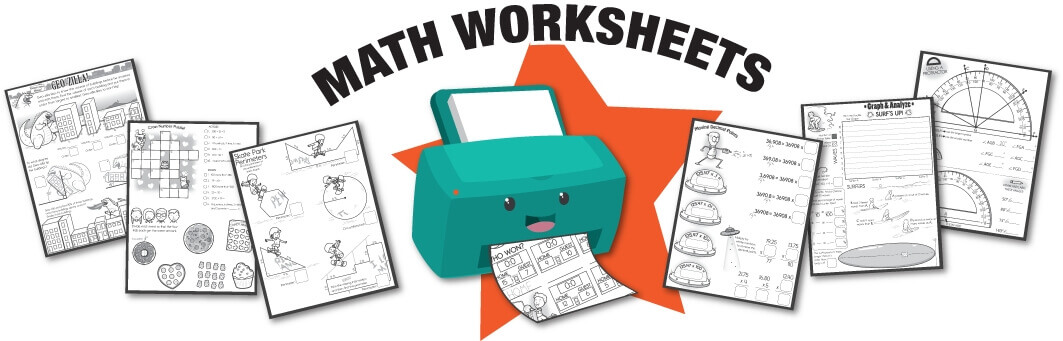# Math Worksheets# Worksheets You'd Want To Print

### Free Printable Math Worksheet Sampler Packs - Need a quick resource for your students to practice their math skills? The sampler packs below are available for free with no login required!Kindergarten Jumbo Math Worksheets PackFirst Grade Jumbo Math Worksheets PackSecond Grade Jumbo Math Worksheets PackThird Grade Jumbo Math Worksheets PackFourth Grade Jumbo Math Worksheets PackFifth Grade Jumbo Math Worksheets PackSixth Grade Jumbo Math Worksheets PackMiddle School Jumbo Math Worksheets Pack

Operations
 Addition      Division      Multiplication Order of Operations      Runaway Math Puzzles      Subtraction

Math Skills
 Addition      Addition and Subtraction      Addition Squares      Algebra      Algebra for Elementary Kids      Algebraic Fractions      Angles      Area      Area and Perimeter      Calendar      Check Writing      Color by Addition      Color by Division      Color by Multiplication      Color by Number      Color by Subtraction      Color by Algebra      Complex Numbers      Comparing Numbers      Conic Sections      Congruent Shapes      Counting      Decimals      Distributive Property      Division      Estimate      Exponents      Factors      Fractions      Functions      Geometry      Glyphs      Graphs      Greater/Less Than      Greatest Common Factor      History of Math      Hundreds Chart      Hundreds Chart Pieces Puzzle      Inequalities      Integers      Irrational Numbers      Least Common Multiple      Left and Right      Linear Equations      Lines and Line Segments      Logarithms      Magic Squares      Math Facts      Math Facts with Fidget Spinners      Math Workbooks      Matrices Mean, Median, Mode, and Range      Measurement      Metric System      Money      Morning Work      Multiplication      Multiplication Squares      Multiplication Table      Number Bonds      Number Line      Number Properties      Odd and Even Numbers      Order of Operations      Ordering Numbers      Ordered Pairs and the Coordinate Plane      Patterns      Perimeter      Percents      Place Value      Polygons      Polynomials      Prime Numbers and Prime Factorization      Printable Books      Probability      Protractor      Quadratic Equations      Rays and Angles      Rounding and Estimation      Ratios      Singapore Math Worksheets      Scientific Notation      Sequences      Shapes      Solid Figures      Square Roots      Skip Counting      Subitizing      Subtraction      Subtraction Squares      Summer Learning and Math Review      Super Silly Squares      Statistics      Symmetry      Temperature      Time and Telling Time      Transformations      Trigonometry      Volume      Word Problems